## 接口分析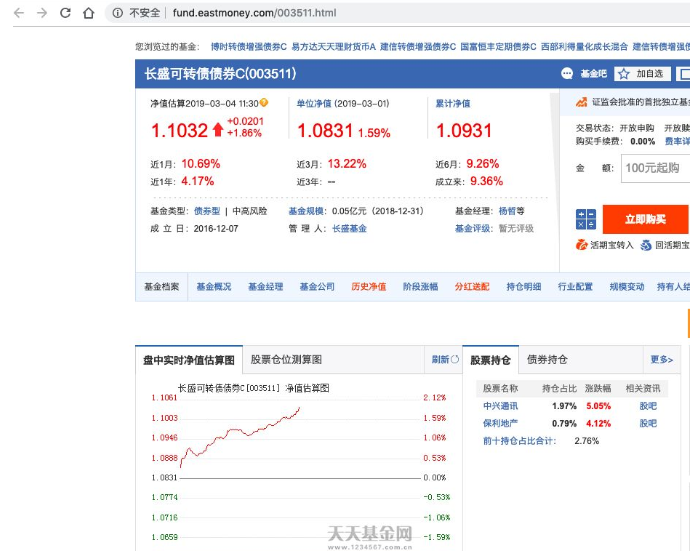## 基金详情

``````http://fund.eastmoney.com/pingzhongdata/003511.js?v=20190304115823
``````

``````http://fund.eastmoney.com/pingzhongdata/
``````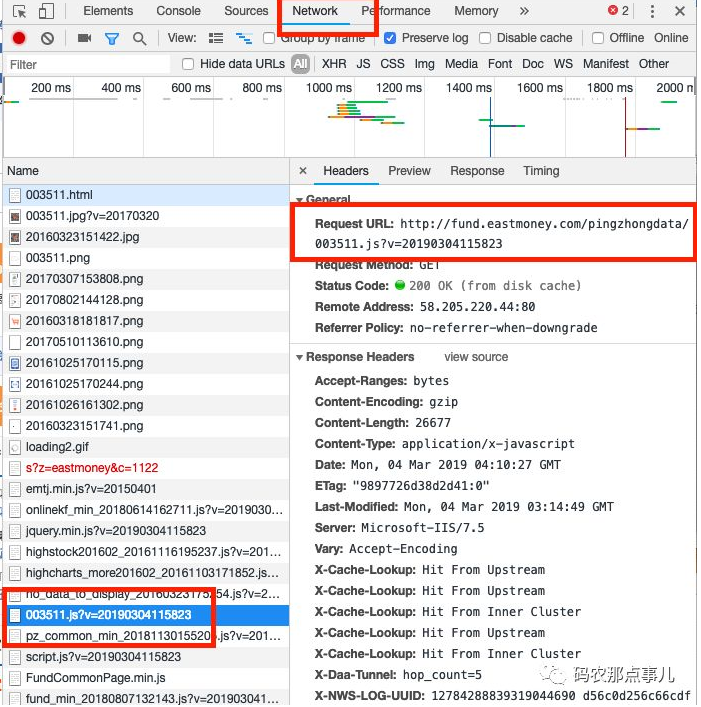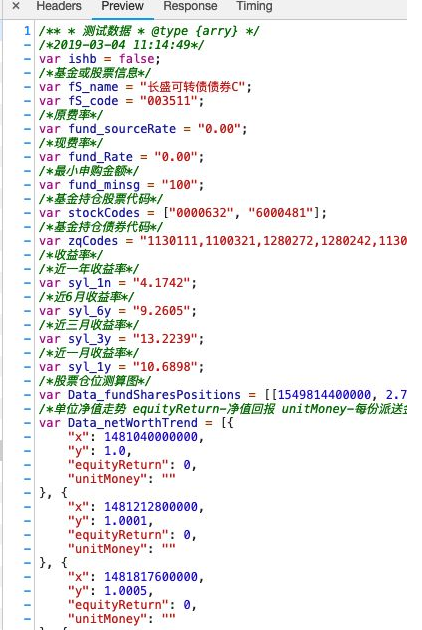``````import requests
import time
import execjs

def getUrl(fscode):
tail = '.js?v='+ time.strftime("%Y%m%d%H%M%S",time.localtime())

def getWorth(fscode):
#用requests获取到对应的文件
content = requests.get(getUrl(fscode))
#使用execjs获取到相应的数据
jsContent = execjs.compile(content.text)
name = jsContent.eval('fS_name')
code = jsContent.eval('fS_code')
#单位净值走势
netWorthTrend = jsContent.eval('Data_netWorthTrend')
#累计净值走势
ACWorthTrend = jsContent.eval('Data_ACWorthTrend')
netWorth = []
ACWorth = []
#提取出里面的净值
for dayWorth in netWorthTrend[::-1]:
netWorth.append(dayWorth['y'])
for dayACWorth in ACWorthTrend[::-1]:
ACWorth.append(dayACWorth)
print(name,code)
return netWorth, ACWorth
``````

``````netWorth, ACWorth = getWorth('003511')
print(netWorth)
``````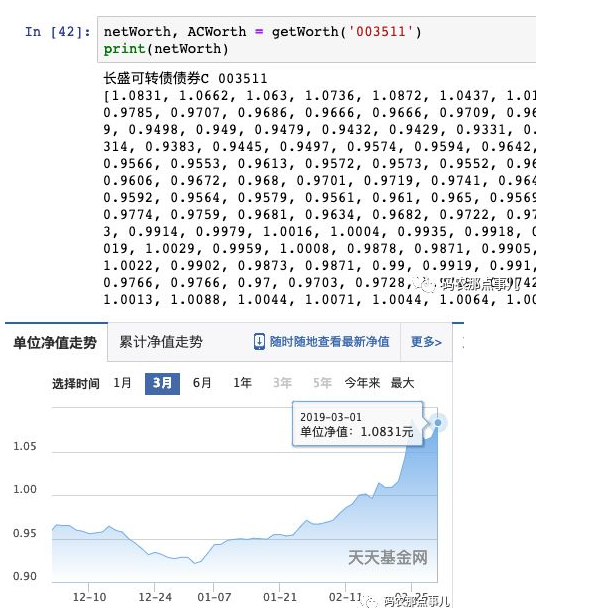``````import matplotlib.pyplot as plt
plt.figure(figsize=(10,5))
plt.plot(netWorth[:60][::-1])
plt.show()
``````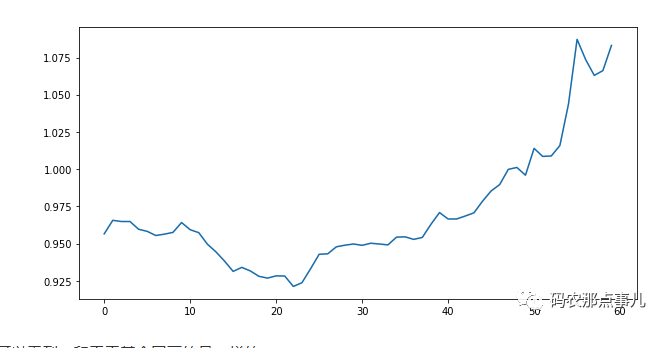``````import requests
import time
import execjs
'''

'''
def getUrl(fscode):
tail = '.js?v='+ time.strftime("%Y%m%d%H%M%S",time.localtime())
# 根据基金代码获取净值
def getWorth(fscode):
content = requests.get(getUrl(fscode))
jsContent = execjs.compile(content.text)
name = jsContent.eval('fS_name')
code = jsContent.eval('fS_code')
#单位净值走势
netWorthTrend = jsContent.eval('Data_netWorthTrend')
#累计净值走势
ACWorthTrend = jsContent.eval('Data_ACWorthTrend')
netWorth = []
ACWorth = []
for dayWorth in netWorthTrend[::-1]:
netWorth.append(dayWorth['y'])
for dayACWorth in ACWorthTrend[::-1]:
ACWorth.append(dayACWorth)
print(name,code)
return netWorth, ACWorth
def getAllCode():
url = 'http://fund.eastmoney.com/js/fundcode_search.js'
content = requests.get(url)
jsContent = execjs.compile(content.text)
rawData = jsContent.eval('r')
allCode = []
for code in rawData:
allCode.append(code)
return allCode
allCode = getAllCode()
netWorthFile = open('./netWorth.csv','w')
ACWorthFile = open('./ACWorth.csv','w')
for code in allCode:
try:
netWorth, ACWorth = getWorth(code)
except:
continue
if len(netWorth)<=0 or len(ACWorth)<0:
print(code+"'s' data is empty.")
continue
netWorthFile.write("\'"+code+"\',")
netWorthFile.write(",".join(list(map(str, netWorth))))
netWorthFile.write("\n")
ACWorthFile.write("\'"+code+"\',")
ACWorthFile.write(",".join(list(map(str, ACWorth))))
ACWorthFile.write("\n")
print("write "+code+"'s data success.")
netWorthFile.close()
ACWorthFile.close()
``````